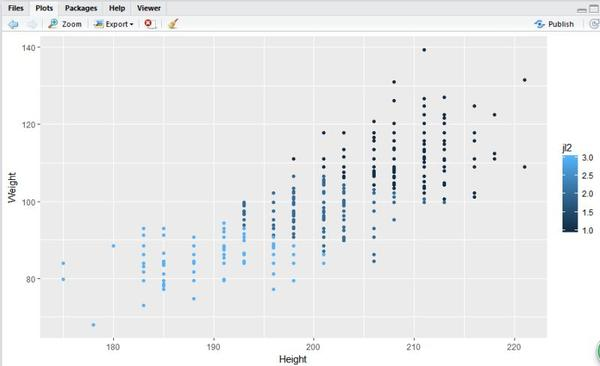# NBA球员体能数据及位置分析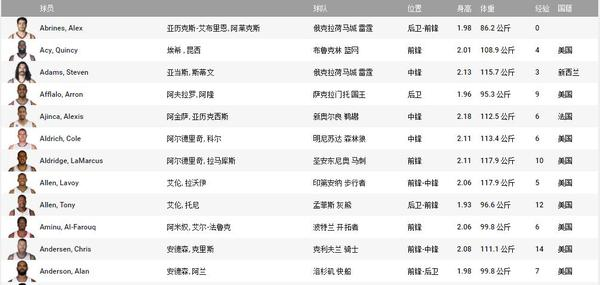1.球员身高分布

``````library(ggplot2)ggplot(hy0209,aes(x=Position,y=Height,col=Position))+geom_dotplot(binaxis = "y",binwidth=0.5,stackdir="center")``````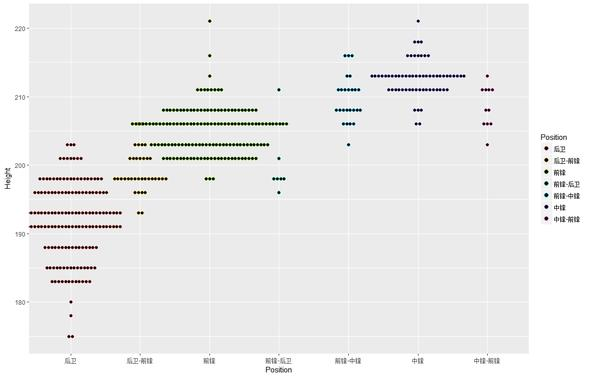``````> hy0209[hy0209\$Height==min(hy\$Height),]
Player         player     team Position Height Weight years country
44 Thomas, Isaiah 托马斯, 以赛亚 凯尔特人     后卫    175   83.9     5    美国
78    Felder, Kay     菲尔德, 凯     骑士     后卫    175   79.8     0    美国``````

``````> hy0209[hy0209\$Height==max(hy\$Height),]Player                   player   team Position Height Weight years  country
146   Marjanovic, Boban         马亚诺维奇, 鲍班   活塞     中锋    221  131.5     1 塞尔维亚
326 Porzingis, Kristaps 波尔津吉斯, 克里斯塔普斯 尼克斯     前锋    221  108.9     1 拉脱维亚``````

``````hy02091<-table(hy0209\$country)
hy02091<-as.data.frame(hy02091)
hy02091<-hy02091[which(hy02091\$Freq>2),]
hy02091<-hy02091[-1,]
ggplot(hy02091,aes(x=Var1,y=Freq))+geom_bar(stat="identity")``````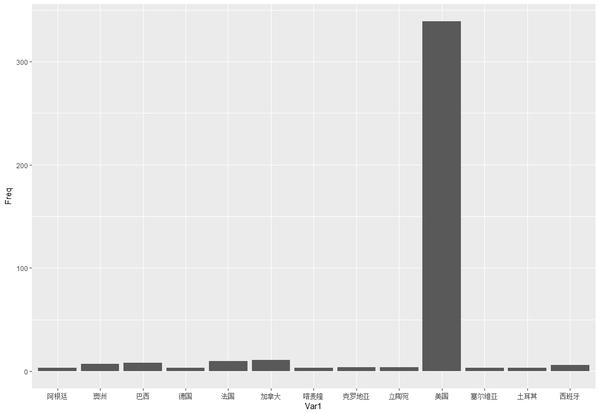NBA拥有来自43个国家的顶级球员，以及4名国籍不明的球员。75.5%的球员来自美国，6.5%来自法国、加拿大、巴西。

``````hy02092<-hy0209[which(hy0209\$Position=="中锋"),]
ggplot(hy02092,aes(x=team))+geom_bar(stat="count")``````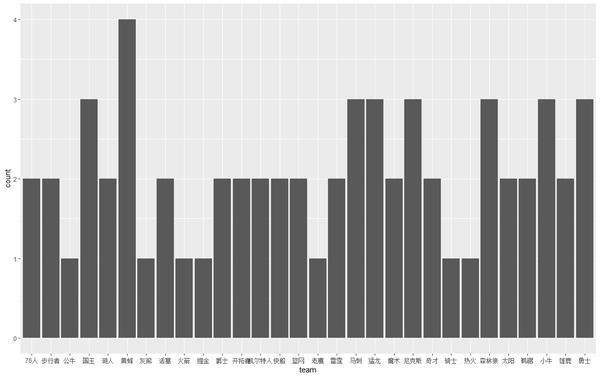1. 地点层面的方差分析``````> lamp<-data.frame(
+ X=hy0210\$Height,
+ A=hy0210\$level)
> lamp.aov<-aov(X ~ A, data=lamp)
> summary(lamp.aov)
Df Sum Sq Mean Sq F value Pr(>F)
A             1  25918   25918    1277 <2e-16 ***
Residuals   447   9073      20
---
Signif. codes:  0 ‘***’ 0.001 ‘**’ 0.01 ‘*’ 0.05 ‘.’ 0.1 ‘ ’ 1``````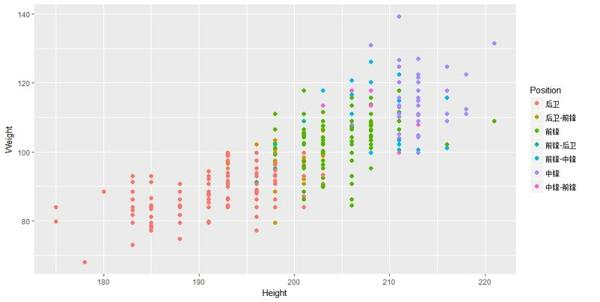``````> cust<-hy[,2:3]
> kmeans_result1<-kmeans(cust,3)
> hy\$jl2<-kmeans_result1\$cluster
> table(hy\$Position,kmeans_result1\$cluster)
> ggplot(hy,aes(x=Height,y=Weight,colour=jl2))+geom_point()``````